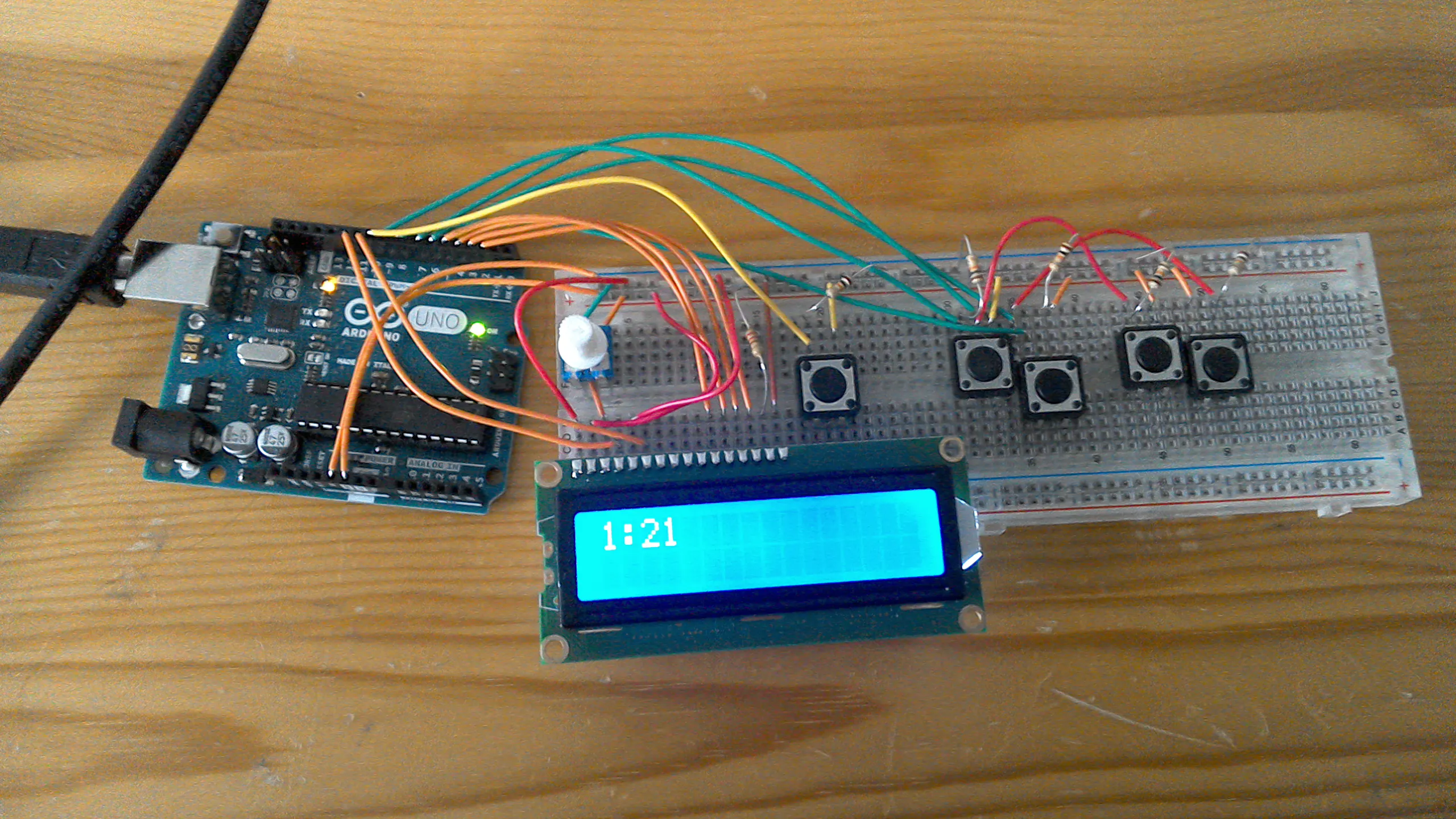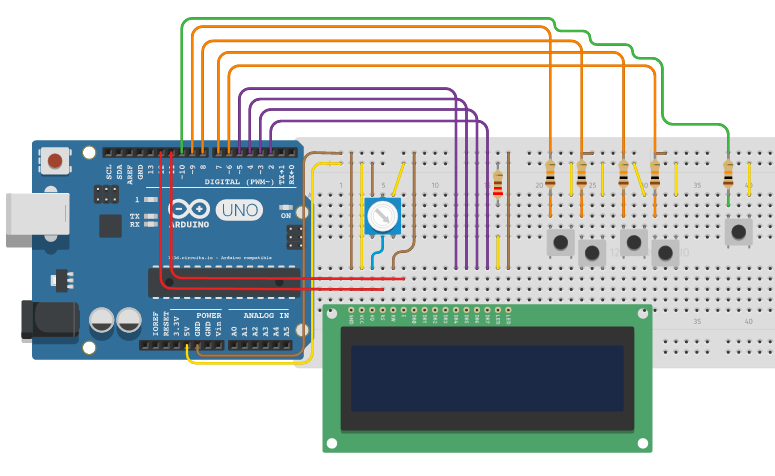Project in progressBasically a digital clock that can have the time changed at the press of a button.

• 14,859 views
• 35 respects

## Apps and online services

My clock works like this, every 60 seconds the minutes go up by 1. every 60 minutes the hours go up by one, and when the hours get to 13, they change to 1. We all know how clocks work. Then if you hold down the change time button for one second, you are able to change the time using the other buttons, as shown in the video.I don't know why I decided to make this, I just thought it would be a fun learning experience. Here is the video of me demonstrating it. Once I eventually get a 3D printer, I will turn this into a fully fledged clock. Sorry about the looks.

## Code

##### My code!C/C++
Here is the code in case anybody wants to try it for themselves.
```#include <LiquidCrystal.h>
//to change time, hold chanage time button for 1 second, then use hour chnage and minute change buttons.

LiquidCrystal lcd(12, 11, 5, 4, 3, 2);
int hour = 12;
int minutes = 0;
int seconds = 0;
int decBtn = 10;
int hrUp = 9;
int hrDwn = 8;
int minUp = 7;
int minDwn = 6;
int hrUpDec = 0;
int hrDwnDec = 0;
int minUpDec = 0;
int minDwnDec = 0;

void setup() {
lcd.begin(16, 2);
pinMode(decBtn, INPUT);
pinMode(hrUp, INPUT);
pinMode(hrDwn, INPUT);
pinMode(minUp, INPUT);
pinMode(minDwn, INPUT);
}

void loop() {
if (digitalRead(hrUp) == HIGH && hrUpDec == 0) {
hrUpDec = 1;
hour = hour + 1;
} else if (digitalRead(hrUp) == LOW) {
hrUpDec = 0;
}
if (digitalRead(hrDwn) == HIGH && hrDwnDec == 0) {
hrDwnDec = 1;
hour = hour - 1;
} else if (digitalRead(hrDwn) == LOW) {
hrDwnDec = 0;
}
if (digitalRead(minUp) == HIGH && minUpDec == 0) {
minUpDec = 1;
minutes = minutes + 1;
} else if (digitalRead(minUp) == LOW) {
minUpDec = 0;
}
if (digitalRead(minDwn) == HIGH && minDwnDec == 0) {
minDwnDec = 1;
minutes = minutes - 1;
} else if (digitalRead(minDwn) == LOW) {
minDwnDec = 0;
}
seconds = 0;
lcd.clear();
if (minutes == 60) {
minutes = 0;
hour = hour + 1;
}
if (minutes < 0) {
minutes = 59;
}
if (hour == 13) {
hour = 1;
}
if (hour < 1) {
hour = 12;
}
lcd.print(hour);
lcd.print(":");
if (minutes < 10) {
lcd.print(0);
}
lcd.print(minutes);
delay(20);
} else if (digitalRead(decBtn) == LOW) {
lcd.clear();
if (seconds == 60) {
seconds = 0;
minutes = minutes + 1;
}
if (minutes == 60) {
minutes = 0;
hour = hour + 1;
}
if (minutes < 0) {
minutes = 59;
}
if (hour == 13) {
hour = 1;
}
if (hour < 1) {
hour = 12;
}
lcd.print(hour);
lcd.print(":");
if (minutes < 10) {
lcd.print(0);
}
lcd.print(minutes);
delay(1000);
lcd.clear();
seconds = seconds + 1;
}
}
```

## Schematics

Just the schematic.#### Simple Arduino Digital Clock Without RTC

Project in progress by Annlee Fores

• 165,047 views
• 155 respects

#### Clock Set Date Time

Project tutorial by Tittiamo

• 97,020 views
• 115 respects

#### Complete Digital Clock Including Alarm and Motion Sensor

Project showcase by LAGSILVA

• 31,590 views
• 97 respects

#### Ternary Digital Clock with Arduino

Project showcase by LAGSILVA

• 8,076 views
• 19 respects

#### How to Make Analog Clock & Digital clock with Led Strip

Project tutorial by DKARDU

• 4,982 views